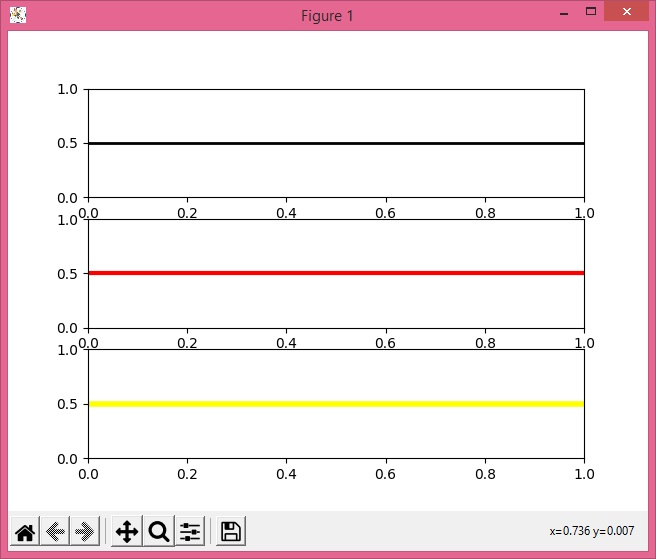# Plotting a horizontal line on multiple subplots in Python using pyplot

MatplotlibPythonData Visualization

To plot a horizontal line on multiple subplots in Python, we can use subplots to get multiple axes and axhline() method to draw a horizontal line.

## Steps

• Create a figure and a set of subplots. Here, we will create 3 subplots.

• Use axhline() method to draw horizontal lines on each axis.

• To display the figure, use show() method.

## Example

from matplotlib import pyplot as plt
fig, (ax1, ax2, ax3) = plt.subplots(3)
plt.rcParams["figure.figsize"] = [7.00, 3.50]
plt.rcParams["figure.autolayout"] = True
ax1.axhline(y=0.5, xmin=0, xmax=3, c="black", linewidth=2, zorder=0)
ax2.axhline(y=0.5, xmin=0, xmax=3, c="red", linewidth=3, zorder=0)
ax3.axhline(y=0.5, xmin=0, xmax=3, c="yellow", linewidth=4, zorder=0)
plt.show()

## Output